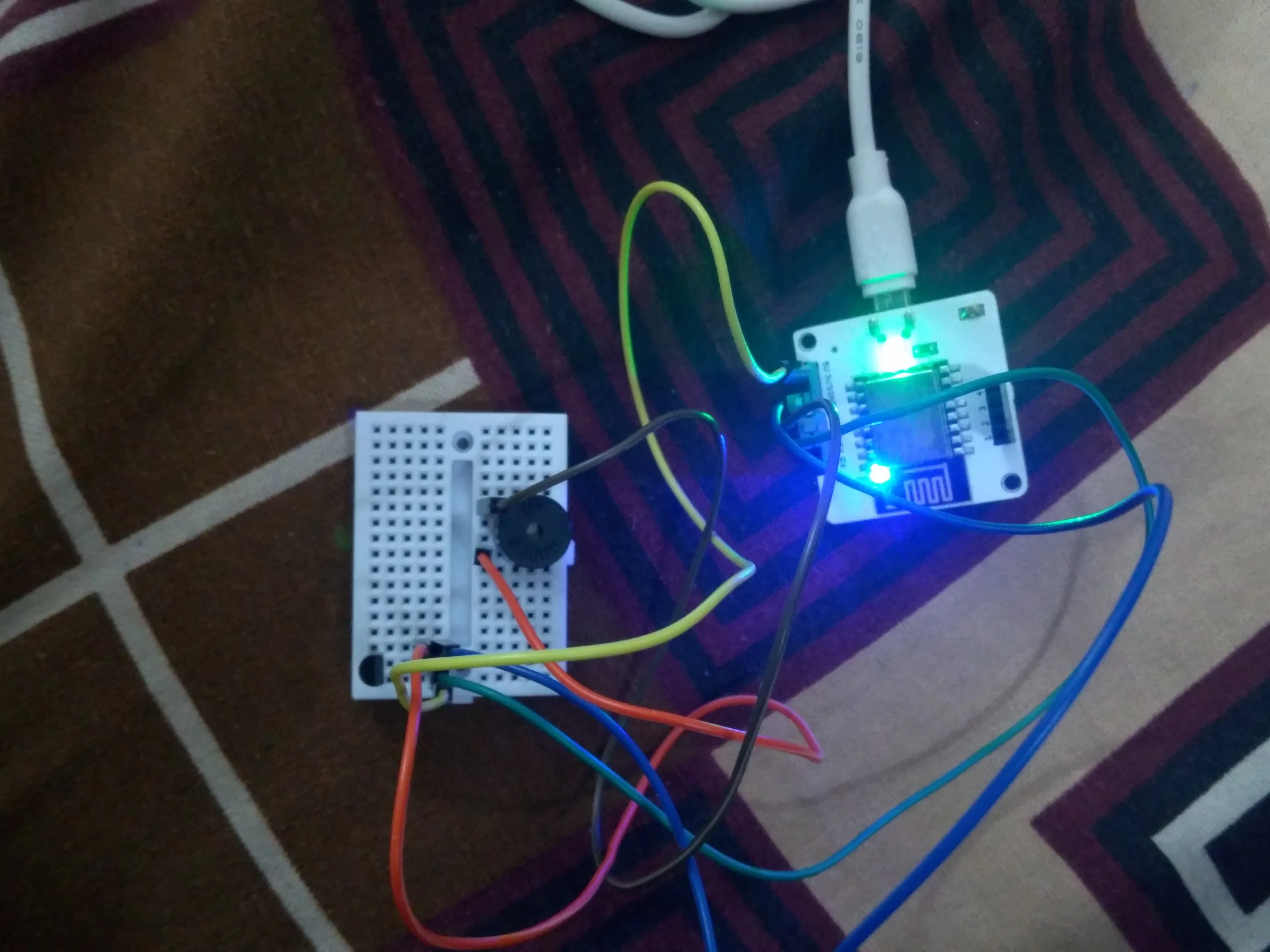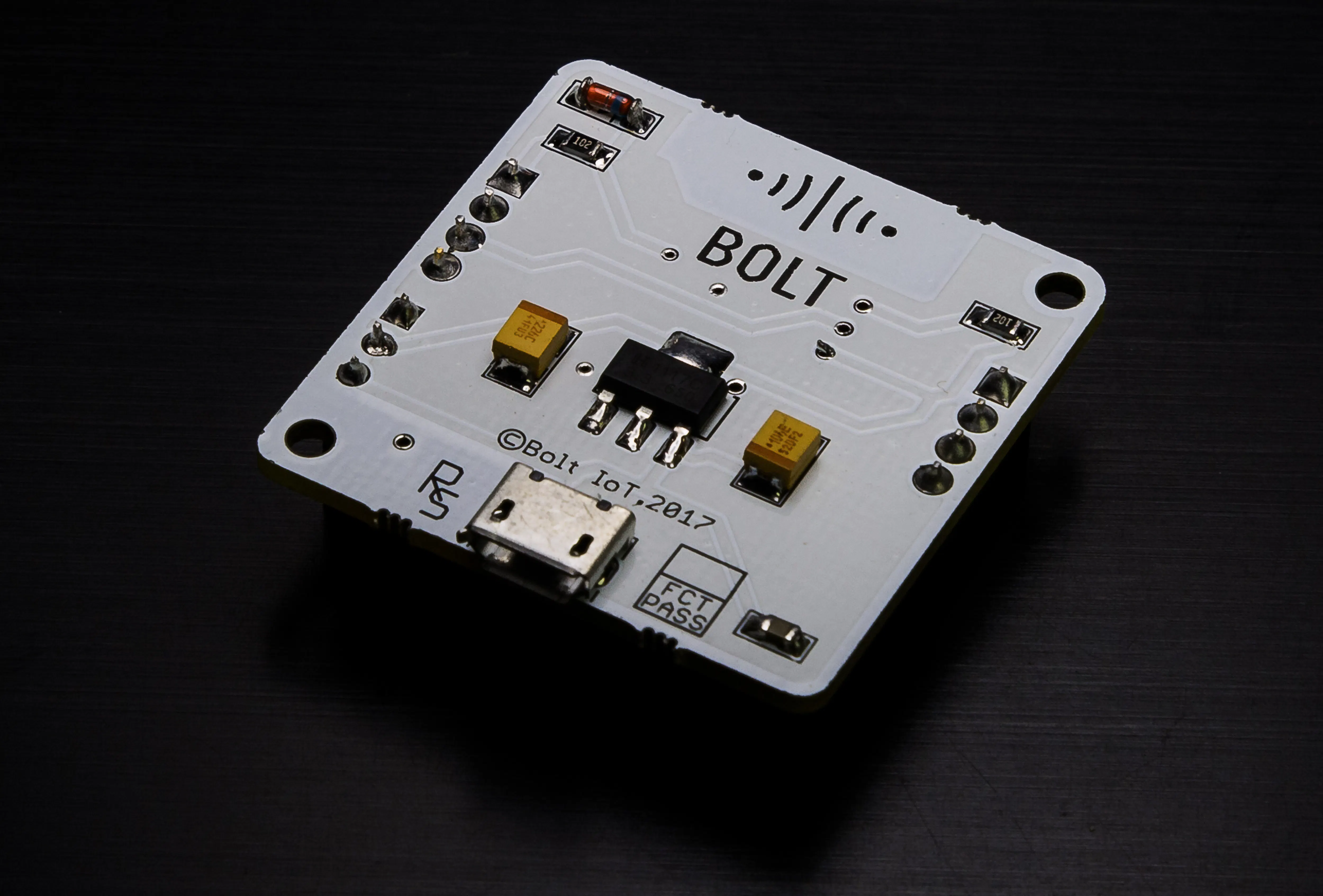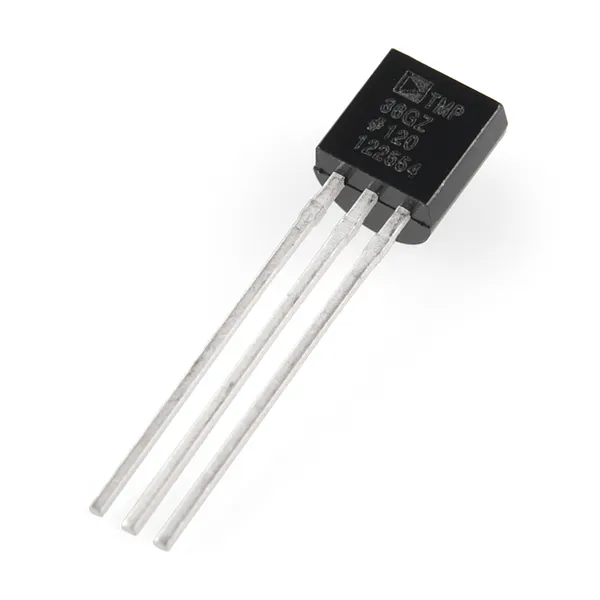Temperature Monitoring System with Buzzer Alert for Anomaly

An automatic temperature monitoring system with an attached alert system. For any change in given condition a buzzer will sound an alert.

BeginnerFull instructions provided2 hours328Things used in this project

Hardware componentsBolt IoT Bolt WiFi Module
×1Temperature Sensor
×1Buzzer
×1×1
 Male/Male Jumper Wires
×5Digilent USB A to Micro-B Cable
×1

Software apps and online servicesSnappy Ubuntu Core

Code

buz.py

Python
from boltiot import Bolt
import json, time, math, statistics
api_key = "c3c7aecf-6aec-45e2-bfca-98158b0cb085"
device_id  = "BOLT3853118"
FRAME_SIZE = 4
MUL_FACTOR = 6
Min_Threshold = 280
Max_Threshold = 250

def compute_bounds(history_data,frame_size,factor):
if len(history_data)<frame_size :
return None

if len(history_data)>frame_size :
del history_data[0:len(history_data)-frame_size]
Mn=statistics.mean(history_data)
Variance=0
for data in history_data :
Variance += math.pow((data-Mn),2)
Zn = factor * math.sqrt(Variance / frame_size)
High_bound = history_data[frame_size-1]+Zn
Low_bound = history_data[frame_size-1]-Zn
return [High_bound,Low_bound]

mybolt = Bolt(api_key, device_id)
history_data = []
while True:
if data['success']!=1:
print("There was an error")
time.sleep(10)
continue
print("The value of temperature is ", data['value'])
sensor_value = 0
z = int(data['value'])
try:
sensor_value = z
except e:
print("There was an error", e)
bound = compute_bounds(history_data,FRAME_SIZE,MUL_FACTOR)
if not bound:
required_data_count=FRAME_SIZE-len(history_data)
print("Not enough data to compute Z-score. Need ",required_data_count," more data points")
history_data.append(int(data['value']))
time.sleep(10)
continue
print("\nZ-score lower bound:", bound)
print("Z-score upper bound", bound)
try:
if sensor_value > bound:
print("The light level increased suddenly. 2 beep buzzer.")
mybolt.digitalWrite('0','HIGH')
time.sleep(1)
mybolt.digitalWrite('0','LOW')
time.sleep(1)
mybolt.digitalWrite('0','HIGH')
time.sleep(1)
mybolt.digitalWrite('0','LOW')
time.sleep(1)
mybolt.digitalWrite('0','HIGH')
time.sleep(3)
mybolt.digitalWrite('0','LOW')
elif sensor_value < bound:
print("The light level decreased suddenly. 1 beep buzzer.")
mybolt.digitalWrite('0','HIGH')
time.sleep(1)
mybolt.digitalWrite('0','LOW')
time.sleep(1)
mybolt.digitalWrite('0','HIGH')
time.sleep(3)
mybolt.digitalWrite('0','LOW')
elif sensor_value < Min_Threshold:
print("The light level is less than Min_Threshold. Normal buzzer.")
mybolt.digitalWrite('0','HIGH')
time.sleep(4)
mybolt.digitalWrite('0','LOW')
elif sensor_value > Max_Threshold:
print("The light level is more than Max_Threshold. Normal buzzer.")
mybolt.digitalWrite('0','HIGH')
time.sleep(4)
mybolt.digitalWrite('0','LOW')
except e:
print("There was an error", e)
history_data.append(sensor_value)
time.sleep(8)

Credits

Hardik Aggarwal

1 project • 0 followers